Checkout JEE MAINS 2022 Question Paper Analysis : Checkout JEE MAINS 2022 Question Paper Analysis :

# Important Questions for Class 12 Chemistry Chapter 9 - Coordination Compounds

Class 12 chemistry important questions with answers are provided here for chapter 9 Coordination Compounds. These important questions are based on CBSE board curriculum and correspond to the most recent Class 12 chemistry syllabus. By practicing these Class 12 important questions, students will be able to quickly review all of the ideas covered in the chapter and prepare for the Class 12 Annual examinations as well as other entrance exams such as NEET and JEE.

Download Class 12 Chemistry Chapter 9 – Coordination Compounds Important Questions with Answers PDF by clicking on the button below.

## Class 12 Coordination Compounds Important Questions with Answers

Q1. Arrange the following complexes in the increasing order of conductivity of their solution:

[Co(NH3)3Cl3], [Co(NH3)4Cl2]CI, [Co(NH3)6]Cl3, [Cr(NH3)5Cl]Cl2

Ionisable ions or molecules exist outside the coordination sphere. When a complex dissolves, it releases more ions, making it more conductive.

[Co(NH3)3Cl3] (1 ion) < [Co(NH3)4Cl2]Cl (2 ions) < [Cr(NH3)5Cl]Cl2 (3 ions) < [Co(NH3)6]Cl3 (4 ions)

Here, the number of ions increases and conductivity increases.

Q2. A coordination compound CrCl, 4H2O precipitates silver chloride when treated with silver nitrate. The molar conductance of its solution corresponds to a total of two ions. Write the structural formula of the compound and name it.

There is chloride ion outside the complex because silver chloride precipitates when treated with silver nitrate. There is only one chloride ion outside the complex since only two ions are generated. As a result, the compound’s structural formula and nomenclature are:

Q3. A complex of the type [M(AA)₂X₂]n+ is known to be optically active. What does this indicate about the structure of the complex? Give one example of such a complex.

In case the given complex is to be optically active, it must be octahedral in nature. This means that ‘A’ must be a bidentate ligand. The complex can have the structure [Pt(en)2Cl2]2+. Only its cis isomer can show optical isomerism.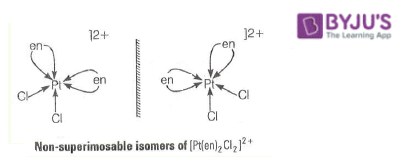Q4. Magnetic moment of [MnCl4]2- is 5.92 BM. Explain why

.

The existence of five unpaired electrons in the d-orbitals of the Mn2+ ion corresponds to a magnetic moment of 5.92 BM. As a result, rather than dsp2, sp3 hybridisation is used. As a result, the tetrahedral structure of the [MnCl4]2– complex will have a magnetic moment of 5.92 BM.

Explanation:

Because the total charge on the coordination sphere is -2 and the charge of the four Cl ligands is -4 in the complex, the oxidation number of the central metal atom Mn will be +2.

Mn ion now has a +2 charge, which means it can only be used once.

These five electrons are all going to be unpaired.

Currently, Magnetic Moment = √n(n+2)

where n is the number of unpaired electrons.

n=5 in this case.

So,

= √5(5+2)

= √35

= 5.916

Magnetic moment = 5.92 BM.

Q5. On the basis of crystal field theory explains why Co (III) forms a paramagnetic octahedral complex with weak field ligands whereas it forms a diamagnetic octahedral complex with strong field ligands.

With weak field ligands, Δo < P. Electronic configuration of Co(III) will be t2g4eg2. Thus, it has 4 unpaired electrons and is paramagnetic. With strong field ligands, Δo > P. Electronic configuration will be t2g6eg0 has no unpaired electron and is diamagnetic.

Q6. Why are low spin tetrahedral complexes not formed?

In a tetrahedral complex, the d-orbital is split too small as compared to octahedral. For the same metal and same ligand Δt = 4/9Δo.

Hence, the orbital energies are not enough to force pairing. As a result, low spin configurations are rarely observed in tetrahedral complexes.

Q7. Give the electronic configuration of the following complexes on the basis of Crystal Field Splitting theory.

[CoF6]3-, [Fe(CN)6]4- and [Cu(NH3)6]2+.

[CoF6]3- = Co3+ = (d)6 = t2g4eg2

[Fe(CN)6]4- = Fe2+ = (d)6 = t2g6eg0

[Cu(NH3)6]2+ = Cu2+ = (d)9 = t2g6eg3

Q8. Explain why [Fe(HO)6]3+ has a magnetic moment value of 5.92 BM whereas [Fe(CN)6]3- has a value of only 1.74 BM.

[Fe(CN)6]3– involves d2sp3 hybridisation with one unpaired electron and [Fe(H2O)6]3+ involves sp3d2 hybridisation with five unpaired electrons. This difference is due to the presence of strong ligand CN and weak ligand H2O in these complexes.

Q9. Arrange the following complex ions in increasing order of crystal field splitting energy (Δo) : [Cr(Cl)6]3–, [Cr(CN)6]3–, [Cr(NH3)6]3+.

The order of increasing Δo value for the complex ions is:

[Cr(Cl)6]3– < [Cr(CN)6]3– < [Cr(NH3)6]3+

This is because of increasing order of the Δo values of the ligands in the spectrochemical series.

Q10. Why do compounds having similar geometry have different magnetic moments?

The presence of weak and strong field ligands in complexes is the reason for this. For example, [CoF6]3- and [Co(NH3)6]3+ are paramagnetic and diamagnetic respectively, because F is a weak field ligand and NH3 is a strong field ligand while both have similar geometry. Because F is a weak field ligand and NH3 is a strong field ligand, the complex will have a low magnetic moment if CFSE is high, and vice versa.

Q11. CuSO4.5H2O is blue in colour while CuSO4 is colourless. Why?

Water acts as a ligand in CuSO4.5H2O, causing crystal field splitting. As a result, in CuSO4.5H2O, a d—d transition is possible and coloured. Crystal field splitting is impossible in anhydrous CuSO4 due to the lack of water (ligand), hence there is no colour.

Q12. Name the type of isomerism when ambidentate ligands are attached to a central metal ion. Give two examples of ambidentate ligands.

Ligands having more than one different binding position are known as ambidentate ligands. For example, SCN has two different binding positions S and N. Coordination compounds containing ambidentate ligands are considered to show linkage isomerism due to the presence of two different binding positions.

e.g., (i) [CO(NH3)5SCN]3+ and (ii) [Fe(NH3)5(NO2)]3+

Q1. Using crystal field theory, draw an energy level diagram, write electronic configuration of the central metal atom/ion and determine the magnetic moment value in the following:

(i) [CoF6]3-, [Co(H2O)6]2+, [Co(CN)6]3-

(ii) [FeF6]3-, [Fe(H2O)6]2+, [Fe(CN)6]4-

[CoF6]3-

Co3+ = 3d6

F ion is a weak field ligand. The value of Δ0 is small (P>Δ0). Electron pairing is not possible.

Number of unpaired electrons = 4

Magnetic moment = √n(n+2) = √4(4+2) = √24 = 4.9BM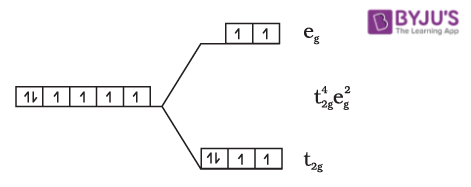[Co(H2O)6]2+

Co2+ = 3d7

H2O is a weak field ligand. The value of Δ0 is small. Electron pairing is not possible.

Number of electrons = 3

√n(n+2) = √3(3+2) = √15 = 3.87BM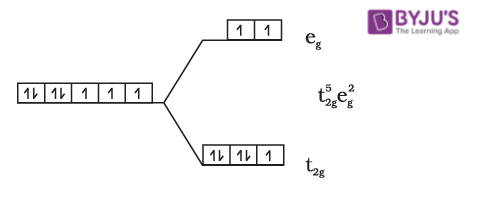[Co(CN)6]3-

Co3+ = 3d6

CN is a strong field ligand. The value of Δ0 is large. Electron pairing is not possible.

Number of unpaired electrons = Zero.

Since there are no unpaired electrons present, the complex is diamagnetic in nature.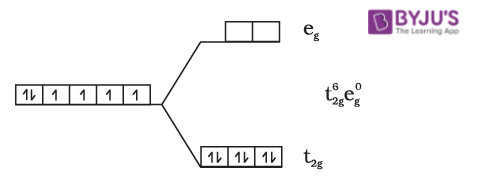[FeF6]3-

Fe3+ = 3d5

F ion is a weak ligand. The value of Δ0 is small (P>Δ0). Electron pairing is not possible.

Number of unpaired electrons = 5

√n(n+2) = √5(5+2) = √3 = 5.92BM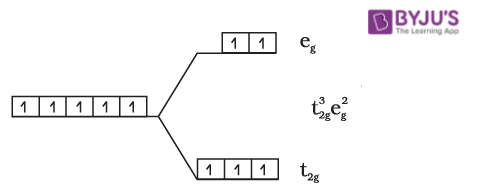[Fe(H2O)6]2+

Fe2+ = 3d6

H2O is a weak field ligand. The value of Δ0 is small (P>Δ0). Electron pairing is not possible.

Number of unpaired electrons = 4

Magnetic moment = √n(n+2) = √4(4+2) = √24 = 4.9BM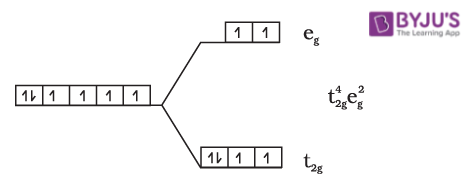[Fe(CN)6]4-

Fe2+ = 3d6

CN ion is a strong field ligand. The value of Δ0 is large (P< Δ0). Electron pairing is possible.

Number of unpaired electrons= zero.

Since there are no unpaired electrons present, the complex is diamagnetic in nature.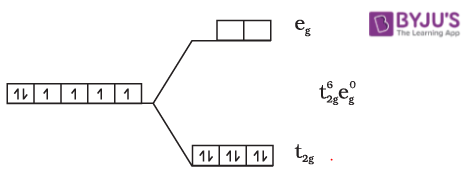Q2. Using valence bond theory, explain the following in relation to the complexes given below:

[Mn(CN)6]3-, [Co(NH3)6]3+, [Cr(H2O)6]3+, [FeCl6]4-

(i) Type of hybridisation.

(ii) Inner or outer orbital complex.

(iii) Magnetic behaviour.

(iv) Spin only magnetic moment value.

[Mn(CN)6]3-

(i) Type of hybridisation = d2sp3 hybridisation

(ii) Inner or outer orbital complex = Inner orbital complex because (n-1)d – orbitals are used.

(iii) Magnetic behaviour = Paramagnetic, as two unpaired electrons are present.

(iv) Spin only magnetic moment value = Spin only magnetic moment (μ) = √2(2+2) = √8 = 2.82BM.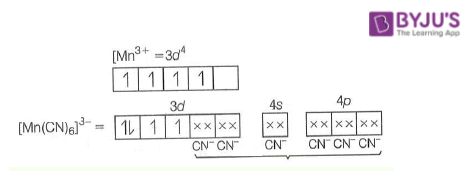[Co(NH3)6]3+

(i) Type of hybridisation = d2sp3 hybridisation

(ii) Inner or outer orbital complex = Inner orbital complex as(n-1)d-orbitals take part.)

(iii) Magnetic behaviour = Diamagnetic (as three paired electrons are present.)

(iv) Spin only magnetic moment value = Spin only magnetic moment (μ) = √0(0+2) = √0 = 0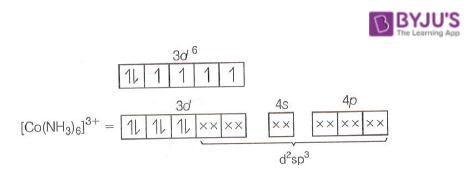[Cr(H2O)6]3+

(i) Type of hybridisation = d2sp3 hybridisation

(ii) Inner or outer orbital complex = Inner orbital complex as(n-1)d-orbitals take part.)

(iii) Magnetic behaviour = Paramagnetic (as three unpaired electrons are present.)

(iv) Spin only magnetic moment value = Spin only magnetic moment (μ) = √3(3+2) = √15 = 3.87BM.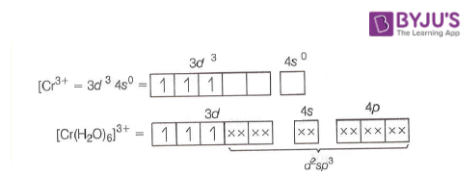[FeCl6]4-

(i) Type of hybridisation = sp3d2 hybridisation

(ii) Inner or outer orbital complex = Outer orbital complex because nd-orbitals are involved in hybridisation.

(iii) Magnetic behaviour = Paramagnetic (because of the presence of four unpaired electrons).

(iv) Spin only magnetic moment value = Spin only magnetic moment (μ) = √4(4+2) = √24 = 4.9BM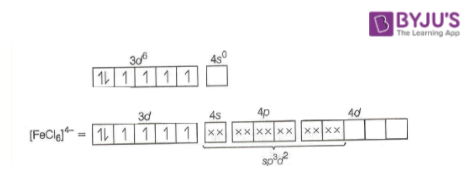Q3. CoSO4CI.5NH3 exists in two isomeric forms ‘A’ and ‘B’. Isomer ‘A’ reacts with AgNO3 to give white precipitate, but does not react with BaCl2. Isomer ‘B’ gives white precipitate with BaCl2 but does not react with AgNO3. Answer the following questions.

(i) Identify ‘A’ and ‘B’ and write their structural formulas.

(ii) Name the type of isomerism involved.

(iii) Give the IUPAC name of ‘A’ and ‘B’.

(i) As isomer A reacts with AgNO3 to give a white precipitate, Cl must be present in the ionisation sphere.

As it does not react with BaCl2, SO42− must be present in the coordination sphere.

Therefore the formula of A = [Co(NH3)5SO4]Cl (coordination no. of Co = 6)

As reactions are reversed for isomer B, formula B = [Co(NH3)5Cl]SO4

(ii) The type of isomerism involved is Ionisation isomerism

(iii) The IUPAC name of

A = Pentaamminesulphatocobalt (III) chloride ,

B = Pentaamminechloridocobalt (III) sulphate.

Q4. What is the relationship between the observed colour of the complex and the wavelength of light absorbed by the complex?

Some of the white light that falls on the complex is absorbed. The lower the wavelength absorbed by the complex, the higher the crystal field splitting energy. The wavelength from the wavelength left behind is used to generate the observed colour of the complex.

When green light is absorbed, for example, the complex appears red. The lower the wavelength absorbed by the complex, the higher the crystal field splitting. The complex’s observed colour is the colour created by the wavelength that was left over.

Assume an octahedral complex with an empty eg level and unpaired electrons in the t2g level in the ground level, according to crystal field theory. If an unpaired electron absorbs light in the blue-green area, it will excite to the eg level, causing the complex to become violet. Crystal field splitting does not occur in the absence of a ligand, and the material is colourless. CuSO4.5H2O is blue in colour, however anhydrous CuSO4 is white.

Q5. Why are different colours observed in octahedral and tetrahedral complexes for the same metal and same ligands?

In the octahedral and tetrahedral fields, the extent of d-orbital splitting differs. In the octahedral and tetra federal fields, the crystal field stabilisation energy is strongly related.

Δt = (4/9)Δo

Where,

Δt = crystal field splitting energy in tetrahedral field

Δo= crystal field splitting energy in octahedral field

Wavelength of light and CFSE (crystal field stabilisation energy) are related to each other by formula

Δo = E = hc/λ

E ∝ 1/λ

For the same metal and ligands, higher wavelengths of light are absorbed in octahedral complexes than in tetrahedral complexes. As a result, many colours can be seen.

## Recommended Videos

#### MCQs on Coordination Compounds#### Isomerism in Coordination CompoundsTest your Knowledge on Important questions class 12 chemistry chapter 9 coordination compounds!